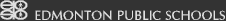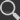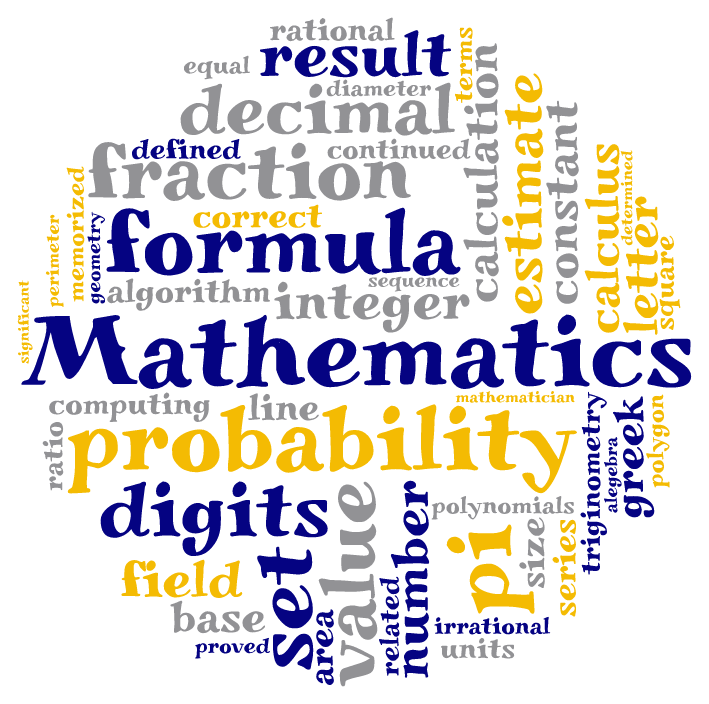# MathematicsThere's a lot more to math than formulas and equations.  In fact you probably use math all the time without even realizing it.

The goal of Mathematics is to prepare you to communicate and reason mathematically, select and use appropriate technologies as tools to solve problems, and connect mathematical ideas to other subjects, concepts and everyday experiences.  It is important to choose courses appropriate to your abilities, interests and goals.

There are three main course sequences in Mathematics. (A graphing calculator (T1-84 plus) is required for all -1 and -2 sequences.)